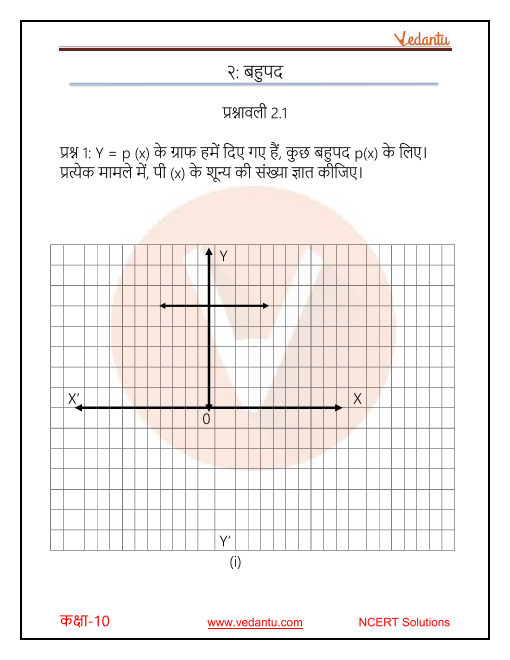# NCERT Solutions for Class 10 Maths Chapter 2 Polynomials in Hindi

## NCERT Solutions for Class 10 Maths Chapter 2 Polynomials in Hindi PDF Download

Download the Class 10 Maths NCERT Solutions in Hindi medium and English medium as well offered by the leading e-learning platform Vedantu. If you are a student of Class 10, you have reached the right platform. The NCERT Solutions for Class 10 Maths in Hindi provided by us are designed in a simple, straightforward language, which is easy to memorize. You will also be able to download the PDF file for NCERT Solutions for Class 10 Maths in Hindi from our website at absolutely free of cost. We, at Vedantu, offer free NCERT Solutions in English medium and Hindi medium for all the classes as well. Subjects like Science, Maths, English will become easy to study if you have access to NCERT Solution for Class 10 Science, Maths solutions, and solutions of other subjects that are available on Vedantu only.

Do you need help with your Homework? Are you preparing for Exams?
Study without Internet (Offline)## NCERT Solutions for Class 10 Maths Chapter 2 Polynomials in Hindi

Chapter-wise NCERT Solutions are provided everywhere on the internet with an aim to help the students to gain a comprehensive understanding. Class 10 Maths Chapter 2 solution Hindi medium is created by our in-house experts keeping the understanding ability of all types of candidates in mind. NCERT textbooks and solutions are built to give a strong foundation to every concept. These NCERT Solutions for Class 10 Maths Chapter 2 in Hindi ensure a smooth understanding of all the concepts including the advanced concepts covered in the textbook.

NCERT Solutions in Hindi medium have been created keeping those students in mind who are studying in a Hindi medium school. These NCERT Solutions for Class 10 Maths Polynomials in Hindi medium pdf download have innumerable benefits as these are created in simple and easy-to-understand language. The best feature of these solutions is a free download option. Students of Class 10 can download these solutions at any time as per their convenience for self-study purpose.

These solutions are nothing but a compilation of all the answers to the questions of the textbook exercises. The answers/solutions are given in a stepwise format and very well researched by the subject matter experts who have relevant experience in this field. Relevant diagrams, graphs, illustrations are provided along with the answers wherever required. In nutshell, NCERT Solutions for Class 10 Maths in Hindi come really handy in exam preparation and quick revision as well prior to the final examinations.

## FAQs on NCERT Solutions for Class 10 Maths Chapter 2 Polynomials in Hindi

1. What are real life applications of Class 10 Mathematics Chapter 2?

Polynomials and its graphical representations which you study in the NCERT Class 10 Maths Chapter 2 have multiple applications in real life. For example, it can be used in designing curves for various products, for modelling and estimating a number of things in Physics, and for cost analysis in economics and finance. With the use of polynomials, you can establish relations between various factors and how one factor affects another.

2. How many exercises, questions and examples are there in Chapter 2 of Class 10 Maths?

There are nine examples and 13 questions spread across four exercises in Chapter 2 of the Class 10 Maths NCERT book. If you have difficulty in understanding this chapter, you should refer to the NCERT Solutions for this chapter available at Vedantu. These solutions are also available on the Vedantu app and are free of cost. You will also get NCERT Solutions Class 10 Maths Chapter 2 in Hindi, for Hindi medium students.

3. What is the difference between quadratic polynomial and cubic polynomial?

The difference between a quadratic polynomial and cubic polynomial is that a quadratic polynomial has a degree of two, whereas the degree of a cubic polynomial is three. A linear polynomial on the other hand has a degree of one. For simplified study material for this chapter, you can download the NCERT Solutions from Vedantu. NCERT Solutions Class 10 Maths Chapter 2 in Hindi is also available for Hindi medium students.

4. Which exercise is optional in Class 10 Maths Chapter 2?

The optional exercise in the NCERT Class 10 Maths Chapter 2 is Exercise 2.4. It is optional because it is not very significant from the perspective of the Class 10 Maths board examination. You can skip it. If you are solving this exercise or any other exercise from this chapter, you can consider taking help from NCERT Solutions for Class 10 Maths Chapter 2 provided by Vedantu.

5. Where to find Class 10 Maths Chapter 2 answers in Hindi?

You can find the best NCERT Solutions Class 10 Maths Chapter 2 in Hindi on Vedantu. The NCERT solutions are even available for download in a PDF format, so you can use this PDF at your speed and convenience. The solution has an explanation of the chapter and solved exercise questions in Hindi. Hindi medium students should use this solution to improve their grades in their Class 10 Maths examination.SHARETWEETSHARESUBSCRIBE